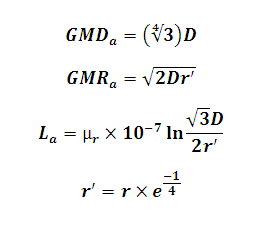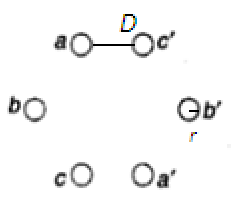# Inductance of Double Circuit Transmission Line Calculator

This Calctown Calculator calculates the inductance of a double circuit transmission line.

Here µ0 = 4p * 10-7. Please enter the RELATIVE permeability accordingly.

m
m
unit

#### Result

m
m
Hwhere

GMDa = geometric mean distance of phase a

GMRa = geometric mean radius of phase a

d = distance between adjacent lines